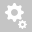##Recently viewed tickets# Crack ratio results in time dependent analysis

Question:

Hi,

I run time dependent material analysis, on output I can find Crack Ratio Results. What does it mean?Thanks

Regards

Hi,

The crack ratio is calculated and included in the results items if you check on the 'Crack' option from the Output Control.Normal crack ratio = allowable tensile stress divided by tensile stress
Inverse crack ratio =  tensile stress divided by allowable tensile stress
Allowable tensile stress is determined from the compressive strength entered in the material data.It is generally used in the heat of hydration analysis.

Regards,
DK Lee

ok thanks, but how Allowable tensile stress is determined from the compressive strength entered in the material data ? which formula?

I think it is used 0.3 x fck ^2/3

Hi,

The tensile strength is calculated using the formula below regardless of selected code.You can define your own Tensile Strength and Elastic Modulus after selecting 'User Defined'.Regards,
DK Lee

ok thanks
Creation date: 1/20/2021 6:55 PM (dklee@midasit.com)      Updated: 1/21/2021 5:29 AM (dklee@midasit.com)